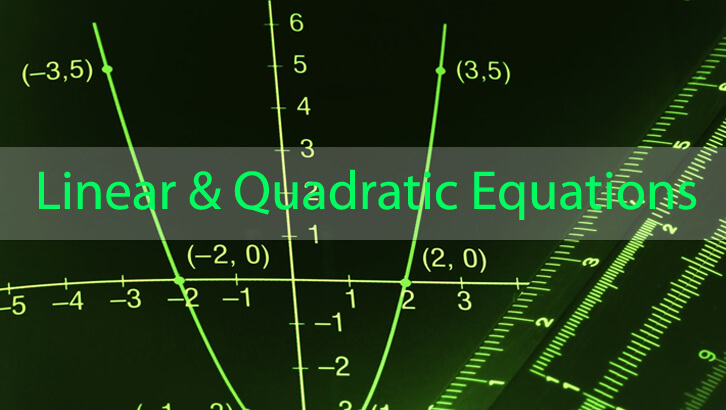# CAT Preparation Online – Simple one from Quadratic Equations

Question

How many integer solutions exist for the equation x2 – 8|x| – 48 =0?

Explanation

One approach is to solve when x > 0 and then solve for x < 0. However, there is a slightly simpler method.

Note that x2 is the same as |x|2, so we can treat the equation as a quadratic in |x|.

Or, |x|2 – 8|x| – 48 = 0
(|x| – 12|) (|x| + 4) = 0
|x| could be 12. |x| cannot be -4.

If |x| could be 12, x can be -12 and 12.

Two possible solutions exist.